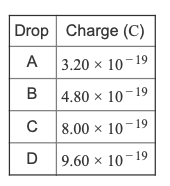# Problem: Part D.  A single electron has a charge of 4.0×10−15 Z . Using zeets and a fictitious unit of mass called the wiggle, w, the charge-to-mass ratio of an electron is 6.4×106 Z / w . What is the mass of an electron in wiggles?Express the mass of an electron in wiggles using two significant figures.In 1909, Robert Millikan performed an experiment involving tiny, charged drops of oil. The drops were charged because they had picked up extra electrons. Millikan was able to measure the charge on each drop in coulombs. Here is an example of what his data may have looked like.

###### FREE Expert Solution
93% (369 ratings)View Complete Written Solution
###### Problem Details

Part D.  A single electron has a charge of 4.0×10−15 Z . Using zeets and a fictitious unit of mass called the wiggle, w, the charge-to-mass ratio of an electron is 6.4×10Z / w . What is the mass of an electron in wiggles?

Express the mass of an electron in wiggles using two significant figures.

In 1909, Robert Millikan performed an experiment involving tiny, charged drops of oil. The drops were charged because they had picked up extra electrons. Millikan was able to measure the charge on each drop in coulombs. Here is an example of what his data may have looked like.